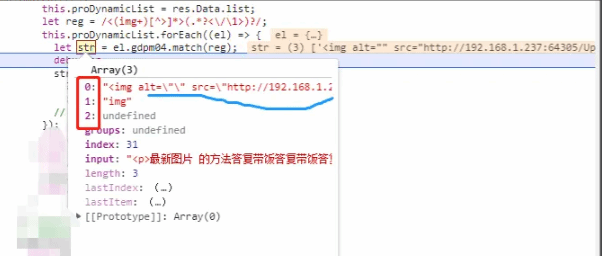# 很讨厌正则，没有原因``````let reg = /<(img+)[^>]*>(.*?<\/\1>)?/;
this.proDynamicList.forEach((el) => {
let str = el.gdpm04.match(reg);
});

````````````let reg = /<(img+)[^>]*>(.*?<\/\1>)?/;
this.proDynamicList.forEach((el) => {
let str = el.gdpm04.match(reg);
str != null
? (el.str = el.gdpm04.replace(str, ""))
: (el.str = el.gdpm04);
// debugger;
});

``````